## Example Questions

2 Next →

### Example Question #51 : Word Translations & Equation Interpretation

At her part-time job, Alexis earns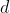dollars per hour and works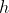hours per week. Which of the following expresses the condition that her total earnings for a week are at least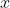dollars?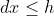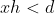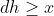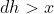Explanation:

What gives this problem its difficulty is its abstraction--all the answer choices are variables--but you can simplify that abstraction in one of two ways:

1) Write out the relationship in words that you know. You know that the amount of money you earn per hour times the number of hours is your total pay. For Alexis, we know that's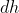. And we know that her total pay is at least (which means greater than or equal to). So by methodically listing out the concepts, not just the variables, we can get to:2) You could also pick simple numbers to set up the math, since most people are much more comfortable with numbers than with variables.  If you were to say that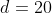(she earns \$20/hour) and that she works 10 hors per week (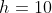), you would know to set up her total pay as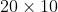. Well, working back to variables, you know that that's. And then if you were to say that that total is at least, that's.

2 Next →

### All SAT Mathematics Resources